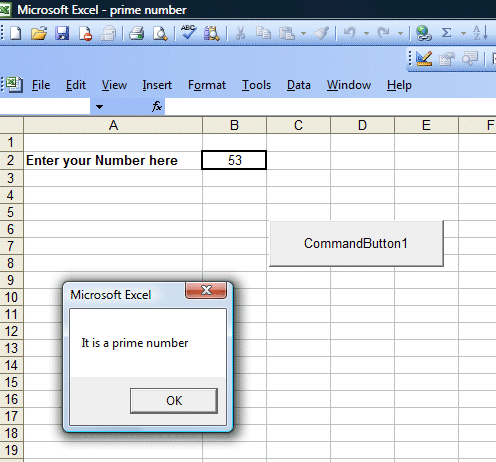Creating a Prime Number Tester

[Back to Excel VBA Sample Code]

This VBA program will test whether a number entered by the user is a prime number or not. Prime number is a number that cannot be divided by other numbers, it includes the number 2 but exclude 1 and 0 and all the negative numbers. Below is the Excel VBA code for the prime number tester:

The Code

Private Sub CommandButton1_Click()

Dim N, D As Single
Dim tag As String
N = Cells(2, 2)

Select Case N
Case Is < 2
MsgBox "It is not a prime number"

Case Is = 2
MsgBox "It is a prime number"

Case Is > 2

D = 2
Do
If N / D = Int(N / D) Then
MsgBox "It is not a prime number"
tag = "Not Prime"
Exit Do
End If
D = D + 1
Loop While D <= N - 1

If tag <> "Not Prime" Then
MsgBox "It is a prime number"
End If
End Select
End Sub

 Explanation: In this program, we use the Select Case  ......End Select statement to determine whether a number entered by a user is a prime number or not. For case 1, all numbers that are less than 2 are not prime numbers. In Case 2, if the number is 2, it is a prime number. In the last case, if the number N is more than 2, we divide this number by all the numbers from 3,4,5,6,........up to N-1, if it can be divided by any of these numbers, it is not a prime number, otherwise it is a prime number. We use a Do......Loop While statement to control the program flow. Besides, we used a tag="Not Prime' to identify the number that is not prime, so that when the routine exits the loop, the label will display the correct answer.

The Interface